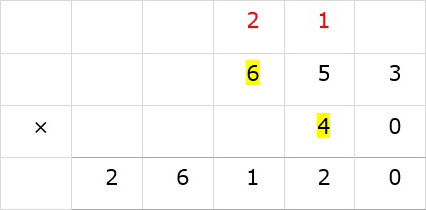# Multiplication with trailing zeros: Problem type 2

Multiply 87 × 60

### Solution

Step 1:

Write the numbers so that 60 is at the bottom. Bring down the trailing zero of 60 as shown.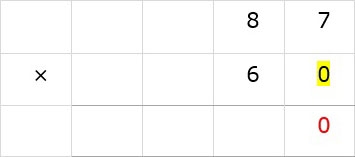Step 2:

Multiply 87 × 6. 6 × 7 = 42. Bring down 2. Carry the 4 to tens column.Step 3:

Multiply 6 × 8 = 48. 48 + 4 = 52. Write 52 as shown.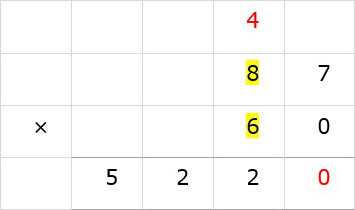Multiply 653 × 40

### Solution

Step 1:

Write the numbers so that 40 is at the bottom.Bring down the trailing zero of 40 as shown.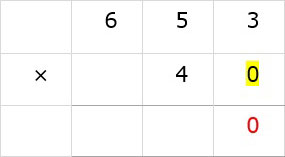Step 2:

Multiply 3 of 653 with 4; 4 × 3 = 12. Bring down 2. Carry the 1 to tens column.Step 3:

Multiply 5 of 653 with 4; 4 × 5 = 20. 20 + 1 = 21. Bring down the 1 and carry 2 to hundreds column.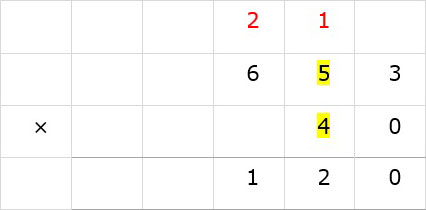Step 4:

Multiply 6 of 653 with 4; 6 × 4 = 24. 24 + 2 = 26. Bring down 26 as shown.